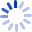# Applied Logistic Regression

Shows how to model a binary outcome variable from a linear regression analysis point of view. Develops the logistic regression model and describes its use in methods for modeling the relationship between a dichotomous outcome variable and a set of covariates. Following establishment of the model there is discussion of its interpretation. Several data sets are the source of the examples and the exercises, and a number of software packages are used to analyze data sets, including BMDP, EGRET, GLIM, SAS, and SYSTAT.

##### Cash for Textbooks